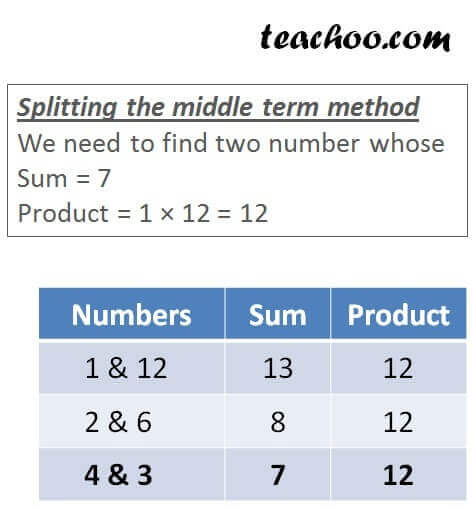Factorisation by middle term

Chapter 2 Class 9 Polynomials
Concept wise

Suppose we want to factorise

x 2 + 7x + 12

We factorise it by splitting the middle term

x 2 + 7x + 12= x 2 + 4x + 3x + 12

= x(x + 4) + 3(x + 4)

= (x + 3)  (x + 4)

So, x 2 + 7x + 12 = (x + 3)  (x + 4)

Learn in your speed, with individual attention - Teachoo Maths 1-on-1 Class